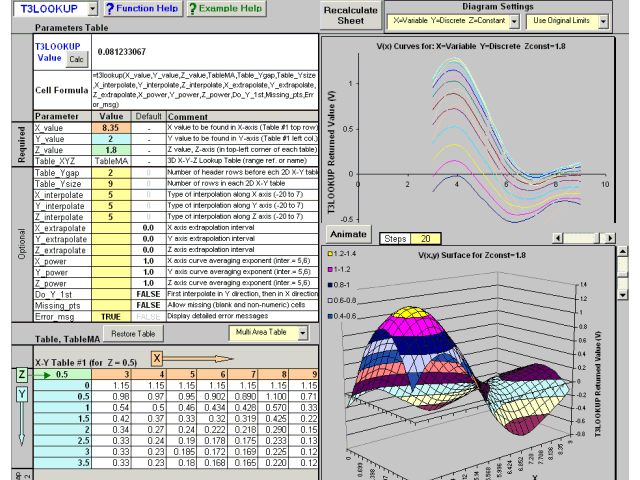# TriLookup Mac 2.2

Program Info

Last Update: 2013-09-20

Release Status: New Release

Size: 0.74 MB

Publisher: Trimill Industrial Systems

OS Support: MAC 68k, Mac PPC, Mac OS 9, Mac OS X, Mac OS X 10.1, Mac OS X 10.2, Mac OS X 10.3, Mac OS X 10.4, Mac OS X 10.5

Category:

Rating
0Publisher's Description

E

xcel add-in with eleven powerful worksheet functions for 1, 2 and 3 variable lookup and interpolation. Interpolation modes include: closest-value, linear, piecewise parabolic or hyperbolic, cubic spline and polynomial up to 20th order. The functions can also handle tables containing missing and invalid data.
Includes functions to fit polynomial curves through a set of X-Y data points and return a table of polynomial curve coefficients, as well as, determine the best fit polynomial curve.
In case of an error, TriLookup functions can either return a standard Excel error code or a detailed text error message, indicating the nature of the error and pointing to the cause of it.
Includes online help and example workbooks. Requires Microsoft Excel 97, 2000, XP or 2003 (Windows) or Excel 98, 2001 or X (Mac).
TVLOOKUP & TVLKP search a multi column table for the lookup value in the lookup column and return a value from the same row of the return column. Can use interpolation and handle missing values.
THLOOKUP & THLKP search a multi row table for the lookup value in the lookup row and return a value from the same column of the return row. Can use interpolation and handle missing values.
T2LOOKUP & T2LKP search a 2D (X-Y) table for X values in top row and Y values in left column, and return a value from the intersecting row and column. Can use interpolation and handle missing values.
T3LOOKUP & T3LKP search a 3D (X-Y-Z) table for X, Y and Z values. Return a value V(x,y,z) from the intersecting row, column and table. Can use interpolation and handle missing values.
TVPOLYDATA & THPOLYDATA Calculate polynomial curve coefficients up to a specified order (using the least squares method) for the X-Y data points given in a table. Also determine the best fit curve.
TPOLY returns the Y value of a polynomial curve for given a X value, polynomial order and an array of polynomial curve coefficients. It can optionally return the value of a derivative.

### Requirements

Excel 98, 2001, X or 2008

### Changes: 2.2

First Macintosh release

English